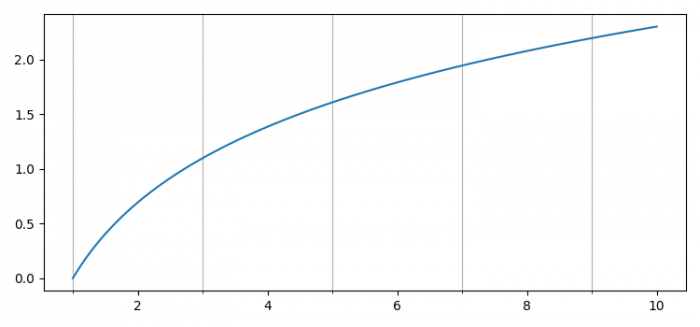# How can I set the location of minor ticks in Matplotlib?

MatplotlibPythonData Visualization

To set the location of the minor ticks in Matplotlib, we can take the following steps −

• Set the figure size and adjust the padding between and around the subplots.
• Create x and y data points using numpy.
• Create a figure and a set of subplots.
• Plot x and y data points using plot() method.
• To locate minor ticks, use set_minor_locator() method.
• To show the minor ticks, use grid(which='minor').
• To display the figure, use show() method.

## Example

import matplotlib.pyplot as plt
import numpy as np
from matplotlib.ticker import AutoMinorLocator

plt.rcParams["figure.figsize"] = [7.50, 3.50]
plt.rcParams["figure.autolayout"] = True

x = np.linspace(1, 10, 100)
y = np.log(x)

fig, ax = plt.subplots()
ax.plot(x, y)
minor_locator = AutoMinorLocator(2)
ax.xaxis.set_minor_locator(minor_locator)
plt.grid(which='minor')

plt.show()

## Output